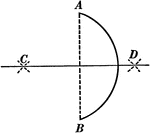### Bisecting an Arc

Illustration used to show how to bisect a given arc.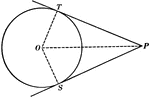### Equal Tangents to Circle Theorem

Illustration used to show that "If two tangents are drawn from any given point to a circle, those tangents…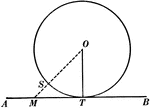### Tangent to Perpendicular Radius Circle Theorem

Illustration used to show that "A tangent to a circle is perpendicular to the radius drawn to the point…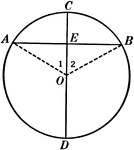### Diameter Perpendicular to a Chord in a Circle

Illustration used to show that "The diameter perpendicular to a chord bisects the chord and also its…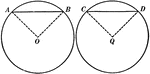### Equal Chords in Equal Circles Theorem

Illustration used to show that "In equal circles, or in the same circle, if two chords are equal, they…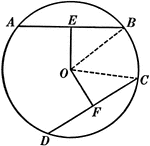### Equal Chords in Equal Circles Theorem

Illustration used to show that "In equal circles, or in the same circle, if two chords are equal, they…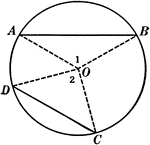### Unequal Chords in Circles Theorem

Illustration used to show that "In equal circles, or in the same circle, if two chords are unequal,…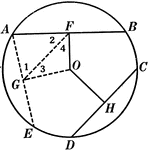### Unequal Chords in Equal Circles Theorem

Illustration used to show that "In equal circles, or in the same circle, if two chords are unequal,…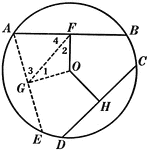### Unequal Chords in Equal Circles Theorem

Illustration used to show that "In equal circles, or in the same circle, if two chords are unequal,…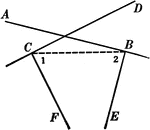### Intersecting Lines Corollary

Illustration used to prove the corollary that "Two lines perpendicular respectively to two intersecting…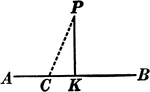### Perpendicular to Line Corollary

Illustration used to prove the corollary that "From a point outside a line there exists only one perpendicular…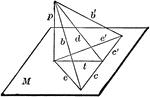### Perpendicular To Line In Plane

"If from the foot of a perpendicular to a plane a straight line is drawn at right angles to any line…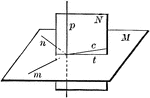### Plane Perpendicular To Line

"All the perpendiculars to a straight line at the same point lie in a plane perpendicular to the line."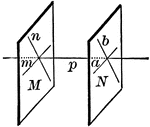### Planes Perpendicular To A Line

"Two planes perpendicular to the same straight line are parallel."### Parallel Lines

"Two straight lines perpendicular to the same plane are parallel."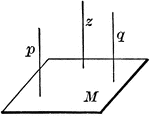### Parallel Lines

"Two straight lines that are parallel to a third are parallel to each other."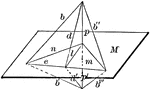### Line Perpendicular To Plane

"If a straight line is perpendicular to each of two straight lines at their point of intersection it…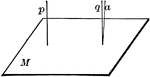### Lines Perpendicular To A Plane

"If one of two parallels is perpendicular to a plane, the other is also."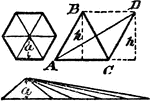### Area Of Regular Polygon Proof

An illustration showing that the area of a regular polygon is equal to the area of a triangle whose…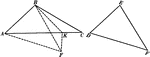### 2 Triangles Theorem

Illustration used to prove that "If two triangles have two sides of one equal respectively to two sides…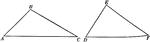### 2 Triangles Theorem

Illustration used to prove that "If two triangles have two sides of one equal respectively to two sides…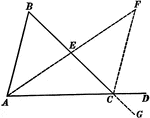### Exterior Angle of Triangle Theorem

Illustration used to prove that "If one side of a triangle is prolonged, the exterior angle formed is…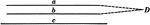### Parallel Lines Theorem

Illustration used to prove that "If two straight lines are parallel to a third straight line, they are…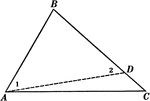### Sides of Triangle Theorem

Illustration used to prove that "If two sides of a triangle are unequal, the angle opposite the greater…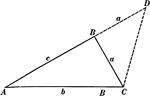### Sides of Triangle Theorem

Illustration used to prove that "The sum of any two sides of a triangle is greater than the third side."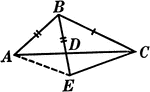### Segments Labeled In A Triangle

Illustration of triangle ABC with BE extended through the triangle at point D. Segment AB is equal to…